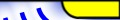Index    Chapter 1    Chapter 2    Chapter 3    Conclusion    Appendix    Matlab Code    Audio DemoOFDM Transceiver code

This code was used for making the practical measurements in section 2.3 of my thesis. This Matlab code allows an OFDM signal to be generated based on an input data file. The data can be random data, a grey scale image, a wave file, or any type of file. The generated OFDM signal is stored as a windows wave file, allowing it to be viewed, listened to and manipulated in other programs. The modified wave file can then be decoded by the receiver software to extract the original data. This code was developed for the experiments that I performed in my honours thesis, and thus has not been fully debugged.

This is the original code developed for the thesis and so has several problems with it. The BER performance given by the simulations is infact Symbol Error Rate.

• Matlab 5 Code for OFDM generation and reception (150kB)
• Matlab 4.2 Code for OFDM generation and reception (160kB)

•  OFDM and CDMA Simulation code

This code was used for generating the performance graphs shown in my thesis. I have updated it to run on Matlab 5.3, however it should still run on Matlab 4. The OFDM simulations are each in a different directory. The cdma code uses generalised code that allows for various different tests. The BER performance given by the simulations is infact Symbol Error Rate.

OFDM

• BER verses Multipath Delay Spread (Figure 17)
• Effect of peak power clipping on OFDM (Figure 18)
• BER verses SNR for OFDM with DBPSK DQPSK, D16PSK (Figure 19)
• Effect of frame synchronisation error on the BER for OFDM (Figure 20)
• CDMA

• BER verse number of users for reverse link(Figure 34)
• BER verses Multipath delay spread (Figure 35)
• Equivalent number of users due to multipath signals (Figure 36)
• BER verses Peak Power Clipping (Figure 37)
• OFDM and CDMA simulation code (95kB)

• Up Publications